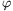Mathematical and Physical Journal
for High Schools
Issued by the MATFUND Foundation
 Already signed up? New to KöMaL?

#Problem A. 415. (December 2006)

A. 415. Let a>b and n be arbitrary positive integers. Prove that n divides(an-bn).

(Proposed by Balázs Strenner, Budapest)

(5 pont)

Deadline expired on January 15, 2007.

Solution. Let d=gcd(a,b), p=a/d and q=b/d. Since pn-qn divides an-bn,(pn-qn) divides(an-bn) and it is sufficient to prove n|(pn-qn). Therefore, it can be assumed that a and b are co-prime.

Note that a and b are relatively prime with an-bn as well.

Consider the multiplicative group of the reduced residue system modulo an-bn. Let c=ab-1. Since

bn<bnc=abn-1<bnc2=a2bn-2<...<bncn-1=an-1b<bncn=an=bn+(an-bn),

the order of element c is exactly n.

By Lagrange's theorem, the order of element c divides the order of the group, therefore n divides(an-bn).

### Statistics:

 9 students sent a solution. 5 points: Gyenizse Gergő, Hujter Bálint, Kisfaludi-Bak Sándor, Kónya 495 Gábor, Korándi Dániel, Lovász László Miklós, Nagy 224 Csaba, Nagy 235 János, Tomon István.

Problems in Mathematics of KöMaL, December 2006